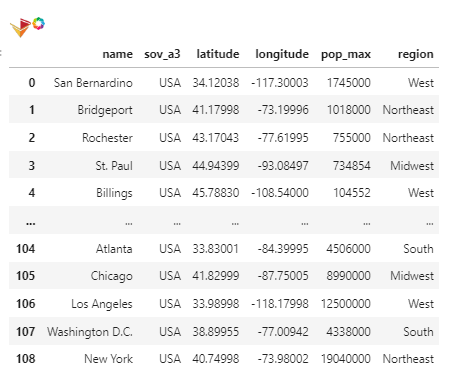# Groupby hvplot

Dear All,

is it possible to add more than 1 group in hvplot
for example with this dataframe:I can manage use 1 groupby “region” but if I want another column to be in groupby it will failed
working code:
from geoviews.element import WMTS
df.hvplot.points(‘longitude’, ‘latitude’, geo=True, color=‘red’, alpha=0.2,groupby=“region”) * WMTS(“https://basemap.nationalmap.gov/arcgis/rest/services/USGSTopo/MapServer/tile/{z}/{y}/{x}”).opts(width=600, height=550)

failed :

How to fix this?

I assume you’ll just need to specify the groups as a list, not as a string.
As you’ve not shared a minimum example code, here a basic, made up example:

``````import hvplot.pandas
import pandas as pd

df = pd.DataFrame(
{'X': [1, 2, 3, 4, 5],
'Y': [1, 2, 3, 4, 5],
'GRP1': ['a', 'a', 'b', 'b', 'c'],
'GRP2': ['aa', 'bb', 'aa', 'bb', 'aa']})

df.hvplot.points('X', 'Y', groupby=['GRP1', 'GRP2'])
``````

Perfect. Thanks @johann